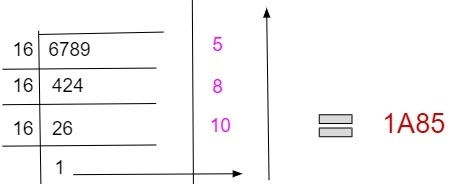# Program for decimal to hexadecimal conversion in C++

Given with a decimal number as an input, the task is to convert the given decimal number into a hexadecimal number.

Hexadecimal number in computers is represented with base 16 and decimal number is represented with base 10 and represented with values 0 - 9 whereas hexadecimal number have digits starting from 0 – 15 in which 10 is represented as A, 11 as B, 12 as C, 13 as D, 14 as E and 15 as F.

To convert a decimal number into a hexadecimal number follow the given steps −

• Firstly divide the given number with the base value of conversion number e.g. dividing 6789 by 16 because we need to convert 6789 into a hexadecimal numbers which have base 16 and then obtain a quotient and store it. If the remainder is between 0-9 store them as it is and if the remainder lies between 10-15 convert them in their character form as A - F
• Divide the obtained quotient with the base value of hexadecimal number which is 16 and keep storing the bits.
• Keep doing right shift to the stored bits
• Repeat the step until the remainder left indivisible

Given below is the pictorial representation of converting a decimal number into a hexadecimal number.## Example

Input-: 6789
Divide the 6789 with base 16 : 6789 / 16 = 5 (remainder) 424(quotient)
Divide quotient with base: 424 / 16 = 8(remainder) 26(quotient)
Divide quotient with base: 26 / 16 = 10(remainder) 1(quotient)
Now reverse the remainder obtained for final hexadecimal value.
Output-: 1A85

## Algorithm

Start
Step 1-> Declare function to convert decimal to hexadecimal
void convert(int num)
declare char arr
set int i = 0
Loop While(num!=0)
Set int temp = 0
Set temp = num % 16
IF temp < 10
Set arr[i] = temp + 48
Increment i++
End
Else
Set arr[i] = temp + 55
Increment i++
End
Set num = num/16
End
Loop For int j=i-1 j>=0 j—
Print arr[j]
Step 2-> In main()
Set int num = 6789
Call convert(num)
Stop

## Example

Live Demo

#include<iostream>
using namespace std;
void convert(int num) {
char arr;
int i = 0;
while(num!=0) {
int temp = 0;
temp = num % 16;
if(temp < 10) {
arr[i] = temp + 48;
i++;
} else {
arr[i] = temp + 55;
i++;
}
num = num/16;
}
for(int j=i-1; j>=0; j--)
cout << arr[j];
}
int main() {
int num = 6789;
cout<<num<< " converted to hexadeciaml: ";
convert(num);
return 0;
}

## Output

IF WE RUN THE ABOVE CODE IT WILL GENERATE FOLLOWING OUTPUT

6789 converted to hexadeciaml: 1A85

Updated on: 18-Oct-2019

1K+ Views